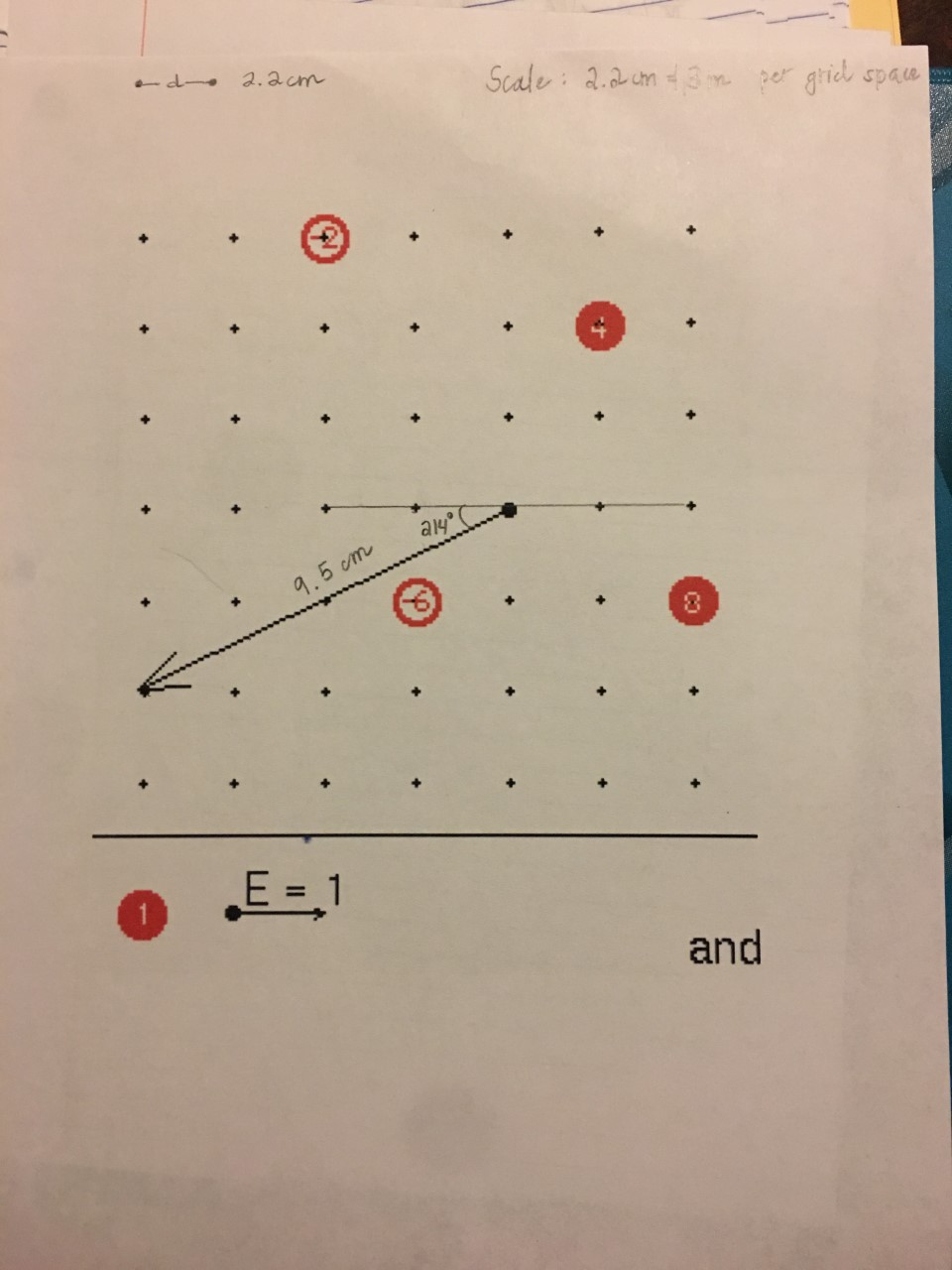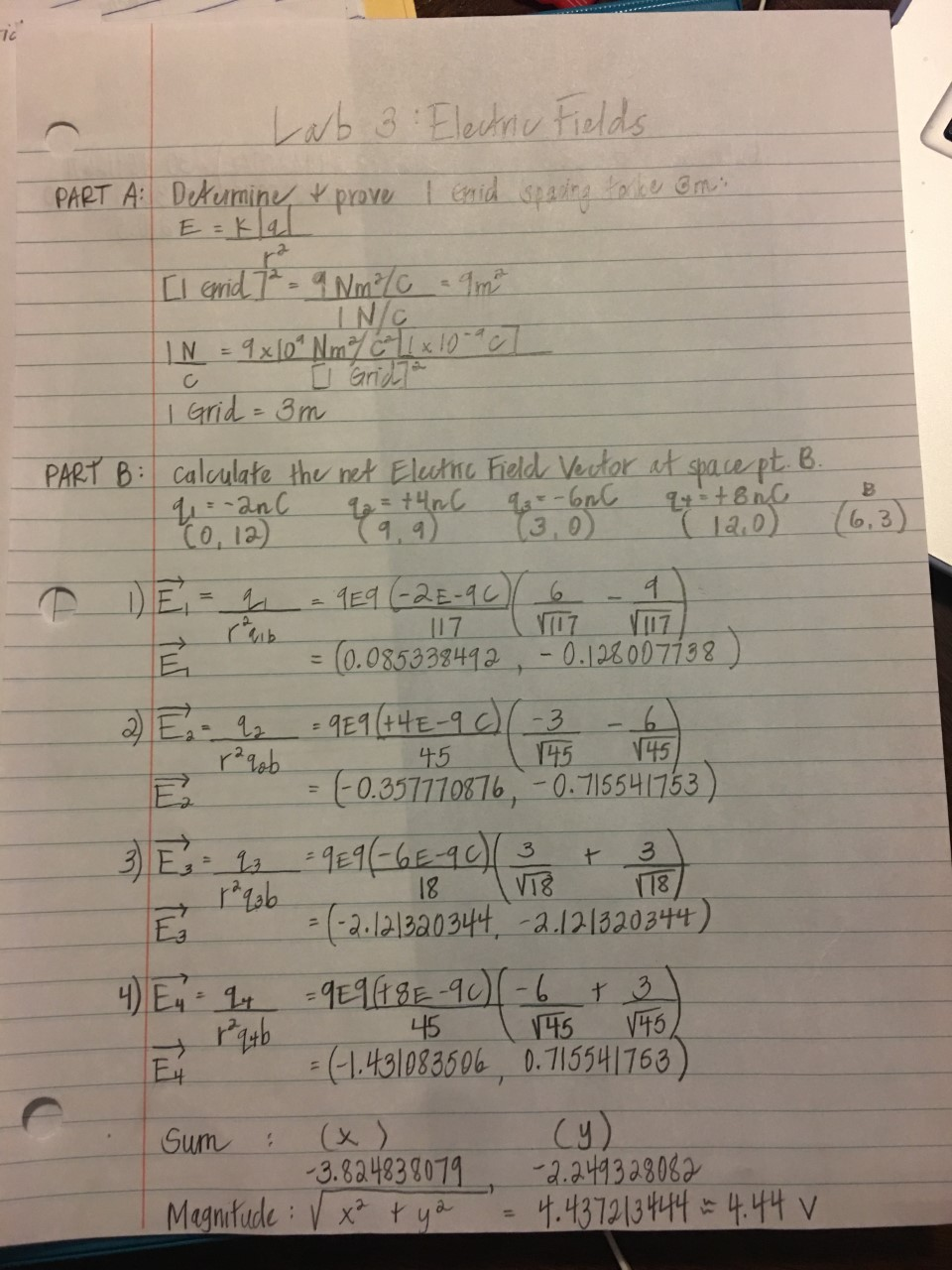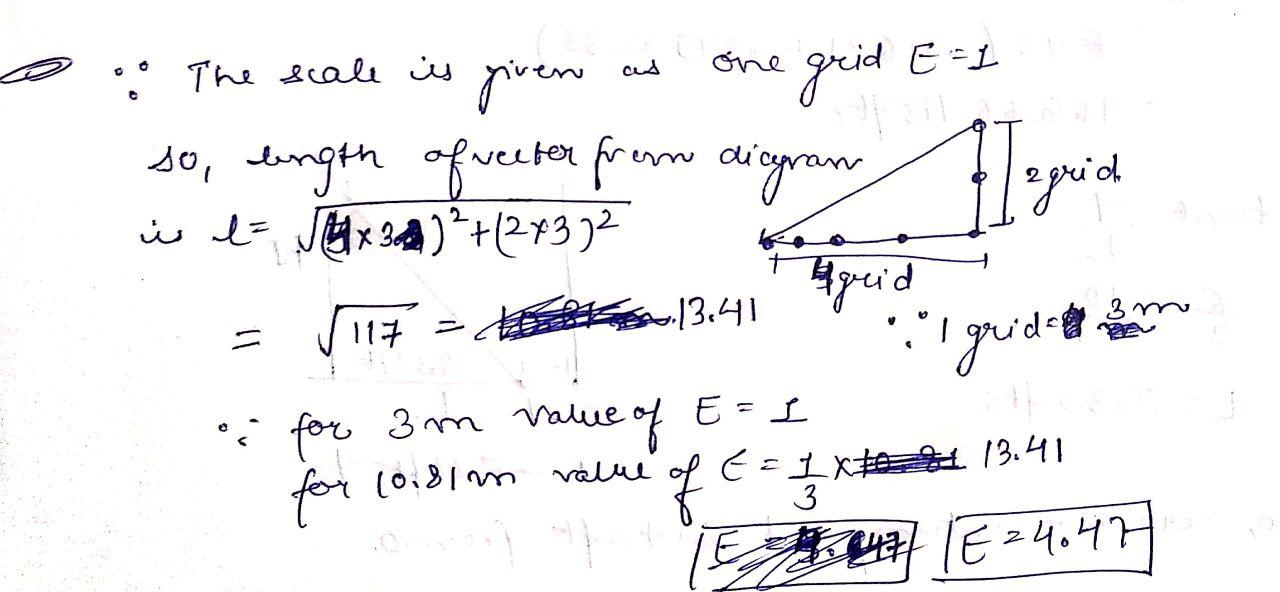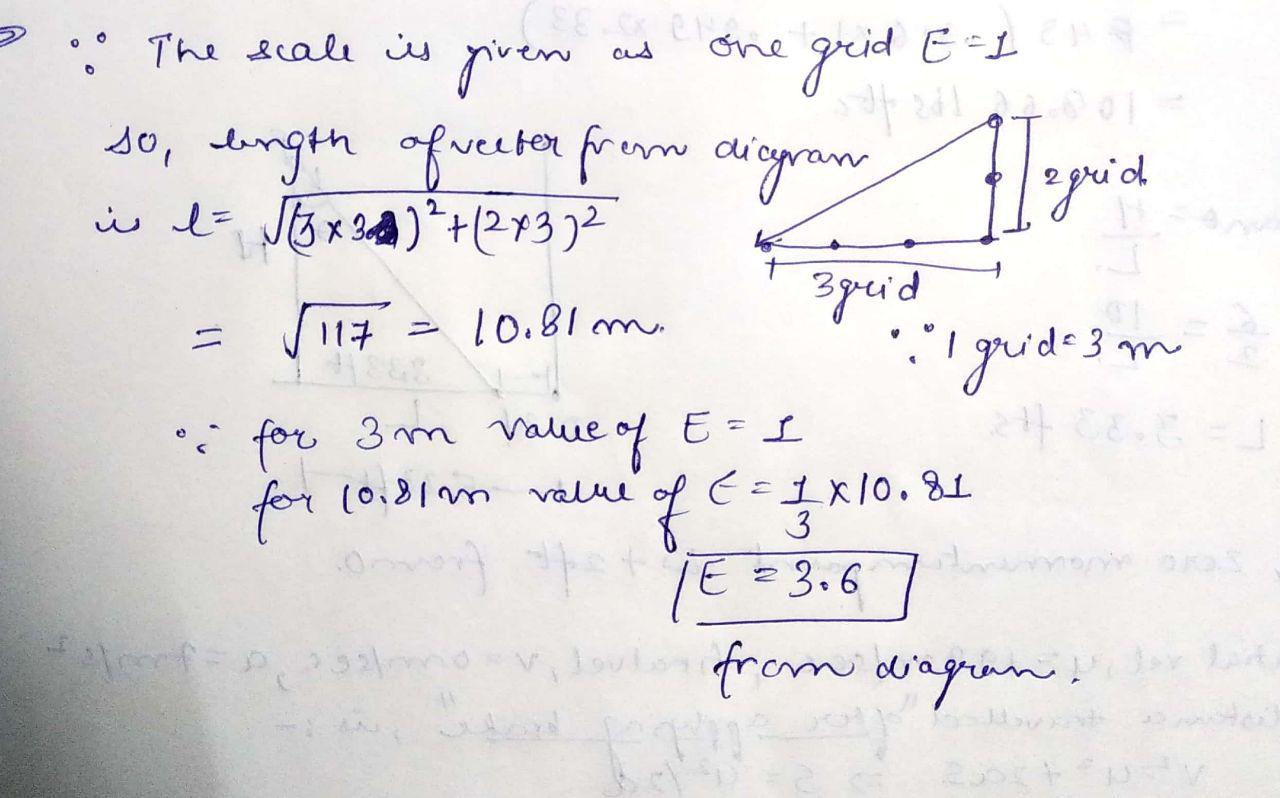# I've done my theoretical calculations (Magnitude E=4.44 V, Direction= 210.5 degrees) but I can't quite remember...I've done my theoretical calculations (Magnitude E=4.44 V, Direction= 210.5 degrees) but I can't quite remember how to scale my vector. My Professor asked each grid space to represent 3 m. I have measured the grid spaces to be 2.2cm and the vector length to be 9.5cm. How do I find E by measuring the vector and using the scale I have found?

ad 2.2cm Scale: 2.2cm t in per gridd space on 9.5cm O E= 1 and
Lab 3: Electric Fields PART A: Determine, & prove I Grid spacing to be on: E=klal [I grid 72 - 9 Nm²/C amt INC IN = 9x[0" Nm 11x10 Gridra c I | Grid = 3m PART B: calculate the net Electric Field Vector at space pt. B.. q = -2nC 2 = t4nc q-6n qr ton Co, 12) (9.9) 3.0 (120) 6.3 E = (0.085338492, -6.122007782) 5338492 -0.1. 7738 TO L r2qob E 45 (145 145 = (-0.357110876, -7. 715541163) E = (-2.121220344 2.121320344) 4) E = 4 = 9E9 (18E-90) (-6 + 3) - 45 1 145 145 (1.431083506 0.715541763) Sum : (x) - -3.824838079 -2.2493 28082 Magnitude : V x² + y2 = 4.43721 3 444 = 4.44 vI agrid 294 & The scale is given as one grid El so, length of weeter from dicgran i to JB x 3cm)*+(2372 = 5117 = 10.81 m. o. for 3 m value of E= 1 for 10.81 m value of t = 7 x 10.81 F E 23.6 7 S almo vilout from diagram i quidem

#### Earn Coin

Coins can be redeemed for fabulous gifts.